# Paracompactness criteria

The following statements are equivalent for an arbitrary completely-regular Hausdorff space(cf. Completely-regular space; Hausdorff space).

1)is paracompact.

2) Each open covering ofcan be refined to a locally finite open covering.

3) Each open covering ofcan be refined to a-locally finite open covering, i.e. an open covering decomposing into a countable collection of locally finite families of sets in.

4) Each open covering ofcan be refined to a locally finite covering (about the structure of the elements of which nothing is assumed).

5) For any open coveringofthere exists an open covering which is a star refinement of.

6) Each open covering ofcan be refined to a conservative covering.

7) For any open coveringofthere exists a countable collection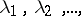of open coverings of this space such that for each pointand for each of its neighbourhoods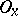there exist a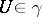and an integersatisfying the condition: Each element of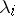intersectingis contained in(i.e. each star of the setrelative tolies in).

8) For any open coveringofthere exists a continuous mapping of the spaceinto some metric spacesubject to the condition: At each point ofthere exists a neighbourhood whose inverse image is contained in an element of.

9) The spaceis collectionwise normal and weakly paracompact.

10) The product ofand any compact Hausdorff space is normal (cf. Normal space).

11)is normal.

12) Every lower semi-continuous multi-valued mapping fromto a Banach space contains a continuous single-valued mapping.

13)admits a uniformity for which the hyperspace of closed sets is complete.

Such a mapping as is posited in 8) is said to realize the covering.

Weakly paracompact spaces are also called metacompact. They are the spaces every open covering of which has a point-finite open refinement.

A family of sets, in particular a covering, is called a conservative family of sets if for every subfamily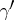of,. Heredenotes the closure of.